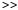Dan Ellis : Research : Music Similarity :

# Artist ID using Matlab, netlab, and uspop2002

We are distributing a large set of precomputed features for 8764 songs from the uspop2002 data set. This page shows a brief example of how to use this data to train a simple classifier - to discriminate between two artists on the basis of a likelihood ratio test between Gaussian Mixture Models (GMMs) trained on each artist. We'll do this within Matlab, and make use of the very nice netlab machine learning toolbox.

We assume that Matlab has the "artist/" directory as its cwd, so we can simply read the HTK files in the subdirectories. We also assume that the standard uspop2002 data files are in "../doc" (as they are on the distribution DVD), and that readhtk.m is available in Matlab's path.

```% Load the master data file describing the datauspop = textread('../doc/uspop2002-aset.txt', '%s');% Reshape...uspop = reshape(uspop, 4, length(uspop)/4);% Now each row describes one track; columns are artist, album, track #, name% Read in the corresponding feature file names for each trackusfiles = textread('../doc/uspop2002-aset-files.txt', '%s');% usfiles(i) is the filename for the track described by uspop(i,:)% Get the indices for all tracks by the Beatles, and by Abbaixbtls = find(strcmp(uspop(1,:), 'beatles'));ixabba = find(strcmp(uspop(1,:), 'abba'));% Concatenate features for the first 10 tracks by Abbada = [];for i = 1:10; da = [da;readhtk(char(usfiles(ixabba(i))))]; end% Similarly for the Beatlesdb = [];for i = 1:10; db = [db;readhtk(char(usfiles(ixbtls(i))))]; end% Train 10 mix GMMs over the 20 dimensional MFCCs, one for each artistndim = 20;nmix = 10;% Initialize and train the 2 GMMsgma = gmm(ndim,nmix,'diag');   % gma is GMM for Abbagmb = gmm(ndim,nmix,'diag');   % gmb is GMM for Beatlesoptions = foptions;     % default optimization optionsoptions(14) = 5;        % 5 iterations of k-means in initializationgma = gmminit(gma,da,options);gmb = gmminit(gmb,db,options);emtions = zeros(1, 18);emtions(14) = 20;       % Number of iterations of EM to do% subsample the training frames by a factor of 10 to speed things upgma = gmmem(gma, da(1:10:end,:), emtions);gmb = gmmem(gmb, db(1:10:end,:), emtions);% Now, evaluate the log-likelihood ratio between the two% models for the first 20 tracks from each artist.  A value% greater than zero indicates classification as Abba, less than% zero indicates the Beatles.  The first 10 tracks in each case% are the training data (should do well!), whereas the next 10% are different.for i = 1:20;  dd = readhtk(char(usfiles(ixabba(i)))); disp(num2str(mean(log(gmmprob(gma,dd)))-mean(log(gmmprob(gmb,dd))))); end
1.7597
1.6639
2.5584
1.3849
3.0646
2.8987
1.1042
3.2112
1.8611
2.3773
1.9904
1.3427
1.9295
1.6319
0.28293
0.2766
2.1931
0.62696
0.55051
-1.4684for i = 1:20;  dd = readhtk(char(usfiles(ixbtls(i)))); disp(num2str(mean(log(gmmprob(gma,dd)))-mean(log(gmmprob(gmb,dd))))); end
-3.2117
-2.3593
-3.6062
-4.2403
-3.9227
-3.3714
-4.1777
-3.6854
-5.5681
-4.4663
-1.952
-4.0578
-2.258
-3.9241
-3.7512
-1.6199
-2.99
-2.0967
-2.8276
-2.2747% Mostly, the Abba files have positive LLRs, and the Beatles have -ve LLRs,% indicating correct classification.  However, the last Abba track is% classified as Beatles - see what it is?usfiles(ixabba(20))
ans =
'abba/Voulez-Vous/06-Does_Your_Mother_Know.htk'% I don't know this track, but maybe it has more Beatles-like instrumentation?
```Last updated: \$Date: 2005/05/28 04:01:07 \$
Dan Ellis <dpwe@ee.columbia.edu>